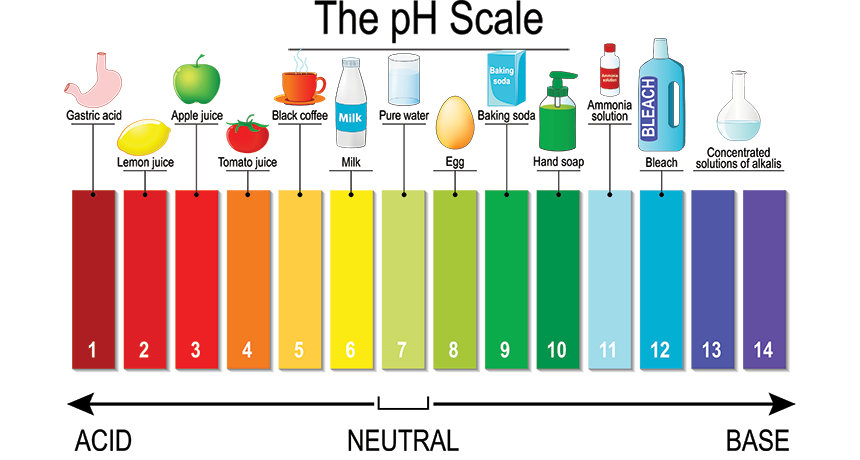# Calculating pH Study Guide

A chemical solution's pH indicates how acidic or alkaline it is.

## INTRODUCTION:

Did you know that the pH of the soil determines the color of hydrangea flowers! The hydrangea's bloom colors are what really set it apart. They range in color from pink to blue, encompassing lavender, violet, purple tints, and green and white.Source

Many hydrangea flowers have a coloration that works as a natural pH indicator for the ground they thrive in. When the shrub is cultivated in acidic soil, the sepals are blue, but when planted in neutral to basic soil, the sepals are red or pink. The hue of the hydrangea blooms shows the pH level of the soil.

There are a lot of indicators to find out whether a given environment is acidic or basic. But are all acidic or basic environments the same? The answer is no! There are different strengths of these too. Some solutions are more acidic than others, whereas some are less acidic. To find out the strength of acidic or basic nature, we define the term "pH", and its value tells us about the strength of acids and bases.

### CALCULATING PH FOR ACIDS AND BASES:

The "potential of hydrogen ion" is referred to as pH. A chemical solution's pH indicates how acidic or alkaline it is. A pH value of seven is neutrality, below seven acidic, and higher than seven basics on the pH scale, which goes from 0 to 14.

The hydrogen ion concentration in a solution is expressed as a negative base ten logarithms. Calculate it by reversing the sign of the log of a specific hydrogen ion concentration.Source

Acids and bases are defined in various ways, but pH relates exclusively to the concentration of hydrogen ions and is used to describe aqueous solutions. When water dissociates, a hydrogen ion and a hydroxide are produced.

Note that [] stands for molarity, M, when computing pH. The molarity of a solution is measured in moles of solute per liter of solution.

The following formula can be used to express the connection between pH and molarity:

``````                               pH=−log[H+]
``````

If you remember the following relation between pH and pOH, you can compute pOH using a pH value:

``````                              pH + pOH = 14
``````

When you're told to find the pH of a base, this is very handy because you'll normally calculate for pOH instead of pH.

## CONCLUSION:

• The pH of a chemical solution reflects how acidic or basic it is.
• The pH of a solution measures the hydrogen ion concentration.
• A pH value of seven is neutrality, below seven acidic, and higher than seven basics on the pH scale.

### FAQs:

1. How do you find H concentration from pH?

If the pH of a solution is established, the hydrogen ion concentrations, [H+], in mol L-1, may be computed.

The negative log (to base 10) of the concentration of hydrogen ions in mol L-1 is stated as pH = -log10[H+].

The following equation can be used to determine [H+] in mol L-1: [H+] = 10-pH

2. Is pH equal to concentration?

The logarithm of the inverse of the concentration of hydrogen ions in gram moles per liter is used to quantify the hydrogen ion concentration. In a neutral solution, the quantities of hydrogen ions (H+) and hydroxyl ions (OH) are comparable. A pH of 7 is considered neutral.

We hope you enjoyed studying this lesson and learned something cool about pH calculation! Join our Discord community to get any questions you may have answered and to engage with other students just like you! Don't forget to download our App to experience our fun, VR classrooms - we promise, it makes studying much more fun! 😎

## SOURCES:

1. Calculating pH. https://www.ck12.org/c/chemistry/calculating-ph/lesson/Calculating-pH-of-Acids-and-Bases-CHEM/. Accessed 27 Jan 2022.
2. Here's How to Calculate pH Values. https://www.thoughtco.com/how-to-calculate-ph-quick-review-606089. Accessed 27 Jan 2022.
3. pH, pOH, and the pH scale. https://www.khanacademy.org/science/chemistry/acids-and-bases-topic/acids-and-bases/a/ph-poh-and-the-ph-scale. Accessed 27 Jan 2022.